# Circle: Diameter, Radius, and Chord Online Quiz

#### Complete Python Prime Pack

9 Courses     2 eBooks

#### Artificial Intelligence & Machine Learning Prime Pack

6 Courses     1 eBooks

#### Java Prime Pack

9 Courses     2 eBooks

Following quiz provides Multiple Choice Questions (MCQs) related to Circle: Diameter, Radius, and Chord. You will have to read all the given answers and click over the correct answer. If you are not sure about the answer then you can check the answer using Show Answer button. You can use Next Quiz button to check new set of questions in the quiz.Q 1 - Find the radius and diameter of the following circle.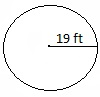### Explanation

Step 1:

Given Radius r = 19 ft

Step 2:

So Diameter = 2r = 2(19) = 38 ft

Step 3:

So, Radius = 19 ft, Diameter = 38 ft

Q 2 - Find the radius and diameter of the following circle.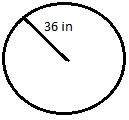### Explanation

Step 1:

Given Radius r = 36 in

Step 2:

So Diameter = 2r = 2(36) = 72 in

Step 3:

So, Radius = 36 in, Diameter = 72 in

Q 3 - Find the radius and diameter of the following circle.### Explanation

Step 1:

Given Diameter d = 44 ft

Step 2:

So Radius r = d/2 = 44/2 = 22 ft

Step 3:

So, Radius = 22 ft, Diameter = 44 ft

Q 4 - Find the radius and diameter of the following circle.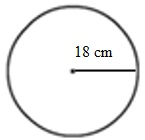### Explanation

Step 1:

Given Radius r = 18 cm

Step 2:

So Diameter = 2r = 2(18) = 36 cm

Step 3:

So, Radius = 18 cm, Diameter = 36 cm

Q 5 - Find the radius and diameter of the following circle.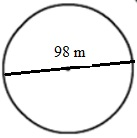### Explanation

Step 1:

Given Diameter d = 98 m

Step 2:

So Radius r = d/2 = 98/2 = 49 m

Step 3:

So, Radius = 49 m, Diameter = 98 m

Q 6 - Find the radius and diameter of the following circle.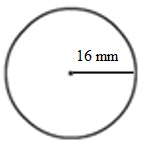### Explanation

Step 1:

Given Radius r = 16 mm

Step 2:

So Diameter = 2r = 2(16) = 32 mm

Step 3:

So, Radius = 16 mm, Diameter = 32 mm

Q 7 - Find the radius and diameter of the following circle.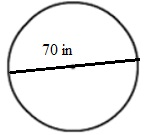### Explanation

Step 1:

Given Diameter d = 70 in

Step 2:

So Radius r = d/2 = 70/2 = 35 in

Step 3:

So, Radius = 35 in, Diameter = 70 in

Q 8 - Identify the radius, diameter, and chord from given circle.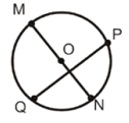### Explanation

Step 1:

The chord is Chord QP

Step 2:

The diameter is Diameter MN

Q 9 - Identify the radius, diameter, and chord from given circle.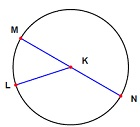### Explanation

Step 1:

Step 2:

The diameter is Diameter MN

Q 10 - Identify the radius, diameter, and chord from given circle.Step 1: